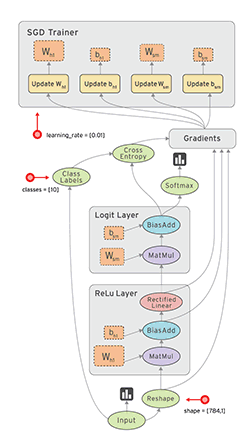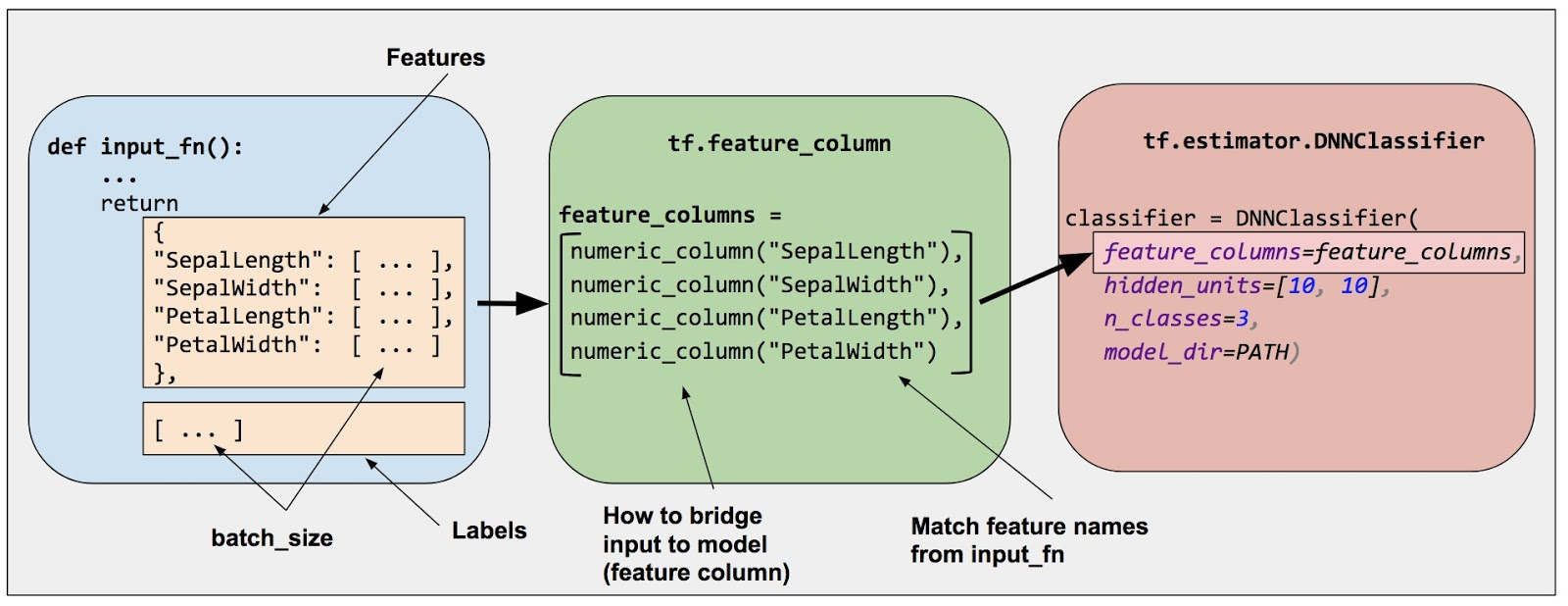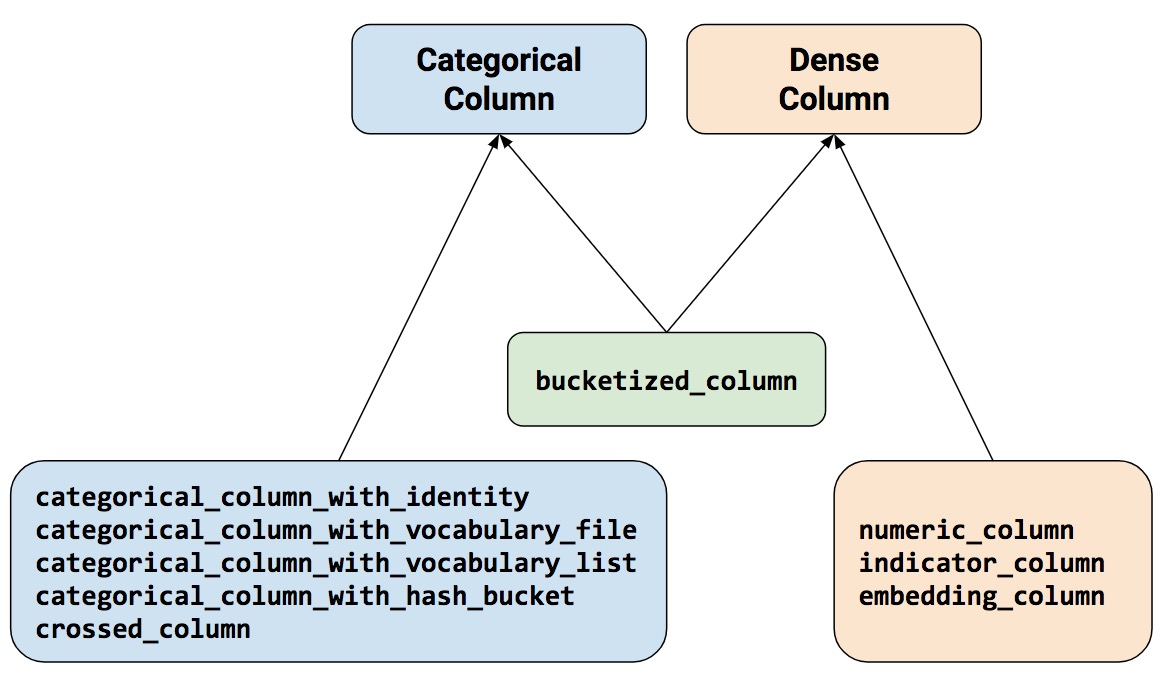This is a simplified version of the official documentation of Tensor Flow.

## Key Abstractions

A metaphor for the relationship between Python and Tensorflow is the relationship between Javascript and HTML. Similarly to HTML, Tensorflow is a framework for representing a certain type of computational abstraction.

## Graph

You might think of TensorFlow Core programs as consisting of two discrete sections:

1. Building the computational graph (a tf.Graph).
2. Running the computational graph (using a tf.Session).img

### What is a computation graph

A computational graph is an abstract concept, it is a series of TensorFlow operations arranged into a graph. The graph is composed of two types of objects.

• Operations (or "ops"): The nodes of the graph. Operations describe calculations that consume and produce tensors.
• Tensors: The edges in the graph. These represent the values that will flow through the graph. Most TensorFlow functions return tf.Tensors.

Important: tf.Tensors do not have values, they are just handles to elements in the computation graph.

Another way to think about it:

TensorFlow programs work by first building a graph of tf.Tensor objects, detailing how each tensor is computed based on the other available tensors and then by running parts of this graph to achieve the desired results.

### What is a tf.Graph()

In practice, a computation graph is represented by tf.Graph(). A tf.Graph contains two relevant kinds of information:

• Graph structure: the nodes and edges of the graph, indicating how individual operations are composed together, but not prescribing how they should be used.
• Graph collections: TensorFlow provides a general mechanism for storing collections of metadata in a tf.Graph.

### Build a tf.Graph()

Most TensorFlow programs start with a dataflow graph construction phase. In this phase, you invoke TensorFlow API functions that construct new tf.Operation (node) and tf.Tensor (edge) objects and add them to a tf.Graph instance.

However, TensorFlow provides a default graph that is an implicit argument to all API functions in the same context.

Some examples

• Calling tf.constant(42.0) creates a single tf.Operation that produces the value 42.0, adds it to the default graph, and returns a tf.Tensor that represents the value of the constant.
• Calling tf.matmul(x, y) creates a single tf.Operation that multiplies the values of tf.Tensor objects xand y, adds it to the default graph, and returns a tf.Tensor that represents the result of the multiplication.
• Executing v = tf.Variable(0) adds to the graph a tf.Operation that will store a writeable tensor value that persists between tf.Session.run calls. The tf.Variable object wraps this operation, and can be used like a tensor, which will read the current value of the stored value. The tf.Variable object also has methods such asassign and assign_add that create tf.Operation objects that, when executed, update the stored value. (See Variables for more information about variables.)
• Calling tf.train.Optimizer.minimize will add operations and tensors to the default graph that calculates gradients, and return a tf.Operation that, when run, will apply those gradients to a set of variables.

High-level APIs such as the tf.estimator.Estimator API manage the default graph on your behalf, and--for example--may create different graphs for training and evaluation.

## Operations

An Operation is a node in a TensorFlow Graph that takes zero or more Tensor objects as input, and produces zero or more Tensor objects as output. Objects of type Operation are created by calling a Python op constructor (such astf.matmul) or tf.Graph.create_op.

For example c = tf.matmul(a, b) creates an Operation of type "MatMul" that takes tensors a and b as input, and produces c as output.

## Tensors

### Tensors

tensor is a generalization of vectors and matrices to potentially higher dimensions. Internally, TensorFlow represents tensors as n-dimensional arrays of base datatypes.

When writing a TensorFlow program, the main object you manipulate and pass around is the tf.Tensor. A tf.Tensor object represents a partially defined computation that will eventually produce a value.

A tf.Tensor has the following properties:

• a data type (float32, int32, or string, for example): Each element in the Tensor has the same data type, and the data type is always known.
• a shape: The shape might be only partially known.

The main types of Tensors are:

With the exception of tf.Variable, the value of a tensor is immutable, which means that in the context of a single execution tensors only have a single value. However, evaluating the same tensor twice can return different values.

See more on Tensors

### Variables

A TensorFlow variable is the best way to represent shared, persistent state manipulated by your program.

Variables are manipulated via the tf.Variable class. A tf.Variable represents a tensor whose value can be changed by running ops on it.

Unlike tf.Tensor objects, a variable maintains state in the graph across multiple calls to run().

Internally, a tf.Variable stores a persistent tensor. Specific ops allow you to read and modify the values of this tensor. These modifications are visible across multiple tf.Sessions, so multiple workers can see the same values for atf.Variable.

Understanding variables is essential to doing deep learning with Tensorflow, because the parameters of your model fall into this category.

When a variable node is first created, it basically stores “null”, and any attempts to evaluate it will result in this exception. We can only evaluate a variable after putting a value into it first.

See more on Variables

### Tensors vs Variables

What's the difference between Tensor and Variable

Variable is basically a wrapper on Tensor that maintains state across multiple calls to run.

A Variable is a Tensor with additional capability and utility. You can specify a Variable as trainable (the default, actually), meaning that your optimizer will adjust it in an effort to minimize your cost function; you can specify where the Variable resides on a distributed system; you can easily save and restore Variables and graphs.

### Tensor-like objects

Many TensorFlow operations take one or more tf.Tensor objects as arguments. For example, tf.matmul takes two tf.Tensor objects, and tf.add_n takes a list of n tf.Tensor objects. For convenience, these functions will accept a tensor-like object in place of a tf.Tensor, and implicitly convert it to a tf.Tensor using the tf.convert_to_tensor method. Tensor-like objects include elements of the following types:

• numpy.ndarray
• list (and lists of tensor-like objects)
• Scalar Python types: bool, float, int, str

You can register additional tensor-like types using tf.register_tensor_conversion_function.

Note: By default, TensorFlow will create a new tf.Tensor each time you use the same tensor-like object. If the tensor-like object is large (e.g. a numpy.ndarray containing a set of training examples) and you use it multiple times, you may run out of memory. To avoid this, manually call tf.convert_to_tensor on the tensor-like object once and use the returned tf.Tensorinstead.

## Feeding

### Placeholders

placeholder is a promise to provide a value later, like a function argument.

We can evaluate this graph with multiple inputs by using the feed_dict argument of the run method to feed concrete values to the placeholders

### Datasets

Placeholders work for simple experiments, but Datasets are the preferred method of streaming data into a model.

Dataset can be used to represent an input pipeline as a collection of elements (nested structures of tensors) and a "logical plan" of transformations that act on those elements.

For more details on Datasets and Iterators see: Importing Data.

## Layers

Layers are the preferred way to add trainable parameters to a graph. Trainable parameters, for example, can be the W in each layer of your Neural Network.

Layers package together both the variables and the operations that act on them.

### Create Layer

To apply a layer to an input, call the layer as if it were a function. For example:

The layer inspects its input to determine sizes for its internal variables. So here we must set the shape of the x placeholder so that the layer can build a weight matrix of the correct size.

### Initialize Layer

Note that this global_variables_initializer only initializes variables that existed in the graph when the initializer was created. So the initializer should be one of the last things added during graph construction.

## Session

A session encapsulates the state of the TensorFlow runtime, and runs TensorFlow operations. If a tf.Graph is like a .py file, a tf.Session is like the python executable.

The session contains a pointer to the global graph, which is constantly updated with pointers to all nodes. That means it doesn’t really matter whether you create the session before or after you create the nodes.

## Estimator

An Estimator is TensorFlow's high-level representation of a complete model. It handles the details of initialization, logging, saving and restoring, and many other features so you can concentrate on your model.

Estimators encapsulate the following actions:

• training
• evaluation
• prediction
• export for serving

An Estimator is any class derived from tf.estimator.Estimator. TensorFlow provides a collection of pre-made Estimators (for example, LinearRegressor) to implement common ML algorithms. Beyond those, you may write your own custom Estimators.

## Feature columns

Think of feature columns as the intermediaries between raw data and Estimators. Feature columns are very rich, enabling you to transform a diverse range of raw data into formats that Estimators can use, allowing easy experimentation.img

Feature columns bridge raw data with the data your model needs.img

Feature column methods fall into two main categories and one hybrid category.

For more details on feature columns, see: https://www.tensorflow.org/guide/feature_columns

https://www.tensorflow.org/guide/low_level_intro

https://www.tensorflow.org/guide/tensors

https://www.tensorflow.org/guide/variables

https://www.tensorflow.org/guide/graphs

https://www.tensorflow.org/api_docs/python/tf/data/Dataset